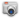Phrases starting with the letter: A B C D E F G H I J K L M N O P Q R S T U V W X Y Z

Previous word: Diagoras
Next word: diagram factor

# Definition of: diagram

Our photo definition of diagram(dīə·gram) noun
1. A line drawing, mechanical plan, or outline, as distinguished from a perspective drawing.
2. A rough projection, map, chart, etc.
3. A figure drawn to aid in demonstrating a geometrical proposition or to illustrate geometrical relations.
4. An outline figure intended to represent any object or area, or to show the relation between parts or places, or to illustrate the value or relations of quantities, forces, etc.
5. A graph.
v.t. ·gramed or ·grammed, ·gram·ing or ·gram·ming

1. To represent by diagram.
2. To illustrate by a diagram. [<Gk. diagramma <dia- across + graphein write]
—di·a·gram·mat·ic
—di

### Most often used phrases:

phase diagram
diagram shows
diagram showing
block diagram
venn diagram
flow diagram
circuit diagram
coxeter dynkin diagram
schematic diagram
dynkin diagram
feynman diagram
voronoi diagram
line diagram
commutative diagram

### 'diagram' used in million biggest domains list by Alexa.com:

clubdediagramas.com
wiringdiagrams21.com
electronic-circuits-diagrams.com
uml-diagrams.org
websequencediagrams.com
dynamicdiagrams.com

### 'diagram' used in other domains:

diagramreklam.com
decisiondiagram.com
waterfalldiagram.com
horsepower-diagram.com
waterwelldiagrams.com
babyteethdiagram.com

### Statistical data

"diagram" has the frequency of use of 0.0001% on city-data.com forum

"diagram" has the frequency of use of 0.001% on en.wikipedia.org.

Phrases starting with the letter: A B C D E F G H I J K L M N O P Q R S T U V W X Y Z